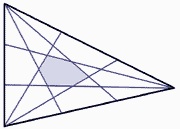# There's an applic- therom for that

Geometry Level 5$\Delta ABC$ has side lengths $4$, $51$ and $53$. Each of its sides are trisected and lines are drawn to each point of trisection from their corresponding angle. By doing this, a hexagon is created in the middle of the triangle. The area of this hexagon can be represented by $p=x(x-1)(x-2)^2+x$ where $x$ is an integer and $p$ is the area of the hexagon. Find $x$.

This problem is similar to one I posted previously but took down. This problem has different lengths and a different solution.

×